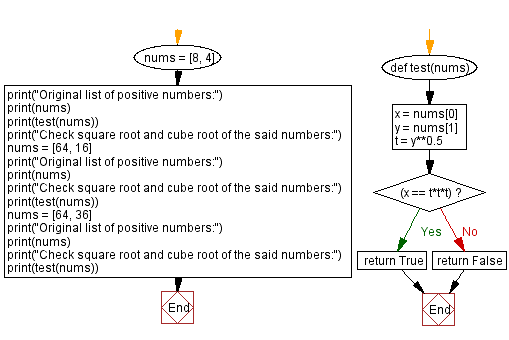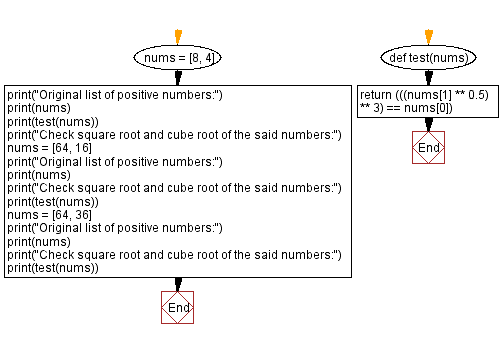﻿ Python - Check square root and cube root of a number

# Python Exercises: Check square root and cube root of a number

## Python Basic - 1: Exercise-146 with Solution

A Python list contains two positive integers. Write a Python program to check whether the cube root of the first number is equal to the square root of the second number.

Sample Data:

([8, 4]) -> True
([64, 16]) -> True
([64, 36]) -> False

Sample Solution-1:

Python Code:

``````def test(nums):
x = nums
y = nums
t = y**0.5
if(x == t*t*t):
return True
else:
return False
nums = [8, 4]
print("Original list of positive numbers:")
print(nums)
print(test(nums))
print("Check square root and cube root of the said numbers:")
nums = [64, 16]
print("Original list of positive numbers:")
print(nums)
print("Check square root and cube root of the said numbers:")
print(test(nums))
nums = [64, 36]
print("Original list of positive numbers:")
print(nums)
print("Check square root and cube root of the said numbers:")
print(test(nums))
``````

Sample Output:

```Original list of positive numbers:
[8, 4]
True
Check square root and cube root of the said numbers:
Original list of positive numbers:
[64, 16]
Check square root and cube root of the said numbers:
True
Original list of positive numbers:
[64, 36]
Check square root and cube root of the said numbers:
False
```

Flowchart:Sample Solution-2:

Python Code:

``````def test(nums):
return (((nums ** 0.5) ** 3) == nums)

nums = [8, 4]
print("Original list of positive numbers:")
print(nums)
print(test(nums))
print("Check square root and cube root of the said numbers:")
nums = [64, 16]
print("Original list of positive numbers:")
print(nums)
print("Check square root and cube root of the said numbers:")
print(test(nums))
nums = [64, 36]
print("Original list of positive numbers:")
print(nums)
print("Check square root and cube root of the said numbers:")
print(test(nums))
``````

Sample Output:

```Original list of positive numbers:
[8, 4]
True
Check square root and cube root of the said numbers:
Original list of positive numbers:
[64, 16]
Check square root and cube root of the said numbers:
True
Original list of positive numbers:
[64, 36]
Check square root and cube root of the said numbers:
False
```

Flowchart:Python Code Editor:

Have another way to solve this solution? Contribute your code (and comments) through Disqus.

What is the difficulty level of this exercise?

Test your Programming skills with w3resource's quiz.

﻿